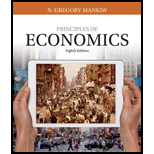# A profit-maximizing firm in a competitive market is currently producing 100 units of output. It has average revenue of $10, average total cost of$8, and fixed cost of $200. a. What is its profit? b. What is its marginal cost? c. What is its average variable cost? d. Is the efficient scale of the firm more than, less than, or exactly 100 units?BuyFindarrow_forward ### Principles of Economics (MindTap C... 8th Edition N. Gregory Mankiw Publisher: Cengage Learning ISBN: 9781305585126 #### Solutions Chapter SectionBuyFindarrow_forward ### Principles of Economics (MindTap C... 8th Edition N. Gregory Mankiw Publisher: Cengage Learning ISBN: 9781305585126 Chapter 14, Problem 7PA Textbook Problem 42 views ## A profit-maximizing firm in a competitive market is currently producing 100 units of output. It has average revenue of$10, average total cost of $8, and fixed cost of$200.a. What is its profit?b. What is its marginal cost?c. What is its average variable cost?d. Is the efficient scale of the firm more than, less than, or exactly 100 units?

Subpart (a):

To determine
Calculate the profit.

### Explanation of Solution

The profit can be calculated by using the following formula:

Profit=(PriceAvarega total cost)×Quantity   (1)

Substitute the value in equation (1) and calculate the profit

Subpart (b):

To determine
The profit, marginal cost, average variable cost, efficient scale of the firm is more or less than or exactly 100 units.

Subpart (c):

To determine
The profit, marginal cost, average variable cost, efficient scale of the firm is more or less than or exactly 100 units.

Subpart (d):

To determine
The profit, marginal cost, average variable cost, efficient scale of the firm is more or less than or exactly 100 units.

### Still sussing out bartleby?

Check out a sample textbook solution.

See a sample solution

#### The Solution to Your Study Problems

Bartleby provides explanations to thousands of textbook problems written by our experts, many with advanced degrees!

Get Started